# 5.4.5 - Logistic Regression - INPUT - Analysis Parameters - Teradata Warehouse Miner

## Teradata Warehouse Miner User Guide - Volume 3Analytic Functions

Product
Release Number
5.4.5
Published
February 2018
Language
English (United States)
Last Update
2018-05-04
dita:mapPath
yuy1504291362546.ditamap
dita:ditavalPath
ft:empty
dita:id
tbj1499730075274
1. On the Logistic Regression dialog box, click INPUT.
2. Click analysis parameters.
Logistic Regression > Input > Analysis Parameters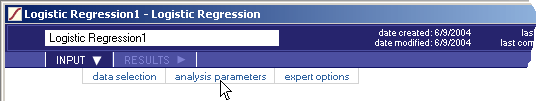3. On this screen, select:
• Regression Options
• Convergence Criterion — The algorithm continues to repeatedly estimate the model coefficient values until either the difference in the log likelihood function from one iteration to the next is less than or equal to the convergence criterion or the maximum iterations is reached. Default value is 0.001.
• Maximum iterations — The algorithm stops iterating if the maximum iterations is reached. The default value is 100.
• Response Value — The value of the dependent variable that will represent the response value. All other dependent variable values will be considered a non-response value.
• Include Constant Term (checkbox) — This option specifies that the logistic regression model should include a constant term.
With a constant, the logistic equation can be thought of as: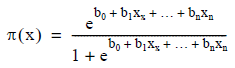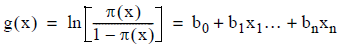Without a constant, the equation changes to: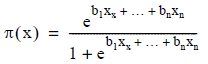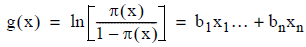The default value is to include the constant term.

• Stepwise Options — If selected, the algorithm is performed repeatedly with various combinations of independent variable columns to attempt to arrive at a final “best” model. The default is to not use Stepwise Regression.
• Step Direction — Selecting “None” turns off the Stepwise option.
• Forward — Option for independent variables being added one at a time to an empty model, possibly removing a variable after a variable is added.
• Forward Only — Option for qualifying independent variables being added one at a time.
• Backward — Option for removing variables from an initial model containing all of the independent variables, possibly adding a variable after a variable is removed.
• Backward Only — Option for independent variables being removed one at a time.
• Criterion to Enter — An independent variable is only added to the model if its W statistic chi-square P-value is less than the specified criterion to enter. The default value is 0.05.
• Criterion to Remove — An independent variable is only removed if its T-statistic P-value is greater than the specified criterion to remove. The default value is 0.05 for each.
• Report Options
• Prediction Success Table — Creates a prediction success table using sums of probabilities rather than estimates based on a threshold value. The default is to generate the prediction success table.
• Multi-Threshold Success Table — This table provides values similar to those in the prediction success table, but based on a range of threshold values, thus allowing the user to compare success scenarios using different threshold values. The default is to generate the multi-threshold Success table.
• Threshold Begin
• Threshold End
• Threshold Increment — Specifies the threshold values to be used in the multi-threshold success table. If the computed probability is greater than or equal to a threshold value, that observation is assigned a 1 rather than a 0. Default values are 0, 1 and .05, respectively.
• Cumulative Lift Table — Produce a cumulative lift table for deciles based on probability values. The default is to generate the Cumulative Lift table.
• (Data Quality Reports) — These are the same data quality reports provided for Linear Regression and Factor analysis. However, in the case of Logistic Regression, the “Sums of squares and Cross Products” or SSCP matrix is not readily available since it is not input to the algorithm, so it is derived dynamically by the algorithm. If there are a large number of independent variables in the model it may be more efficient to use the Build Matrix function to build and save the matrix and the Linear Regression function to produce the Data Quality Reports listed in Data Quality Reports.
• Variable Statistics — This report gives the mean value and standard deviation of each variable in the model based on the derived SSCP matrix.
• Near Dependency — This report lists collinear variables or near dependencies in the data based on the derived SSCP matrix.
• Condition Index Threshold — Entries in the Near Dependency report are triggered by two conditions occurring simultaneously. The one that involves this parameter is the occurrence of a large condition index value associated with a specially constructed principal factor. If a factor has a condition index greater than this parameter’s value, it is a candidate for the Near Dependency report. A default value of 30 is used as a rule of thumb.
• Variance Proportion Threshold — Entries in the Near Dependency report are triggered by two conditions occurring simultaneously. The one that involves this parameter is when two or more variables have a variance proportion greater than this threshold value for a factor with a high condition index. Another way of saying this is that a ‘suspect’ factor accounts for a high proportion of the variance of two or more variables. This parameter defines what a high proportion of variance is. A default value of 0.5 is used as a rule of thumb.
• Detailed Collinearity Diagnostics — This report provides the details behind the Near Dependency report, consisting of the “Eigenvalues of Unit Scaled X’X”, “Condition Indices” and “Variance Proportions” tables.